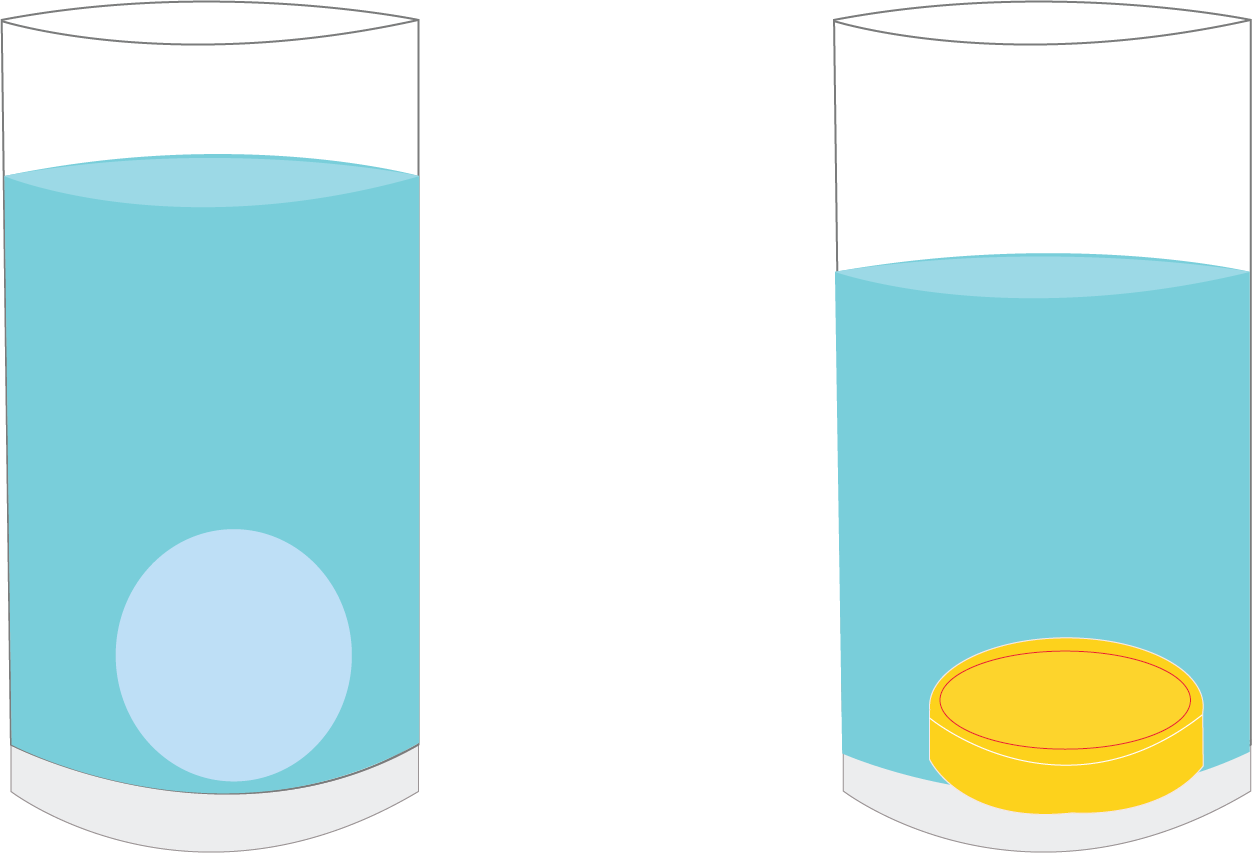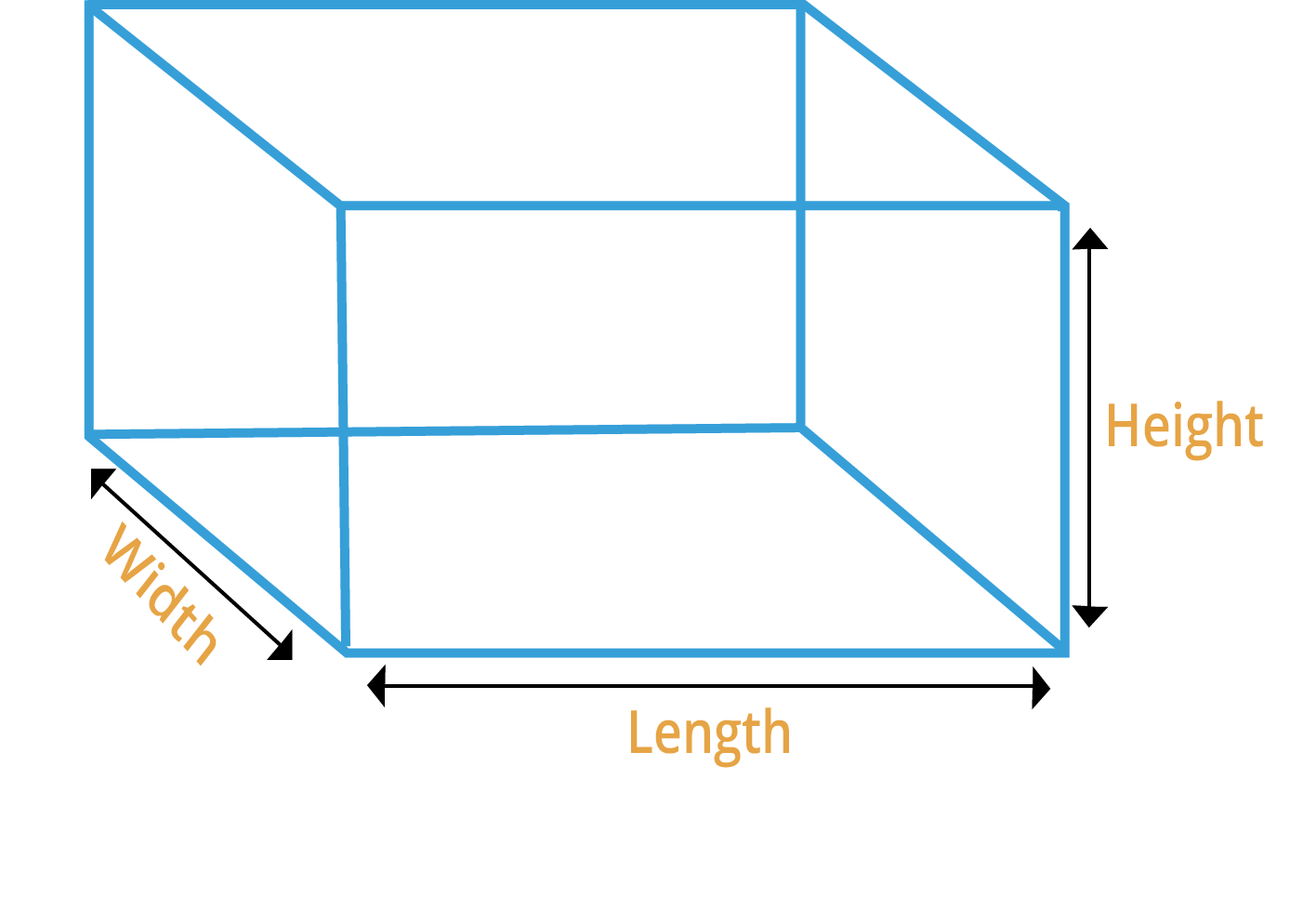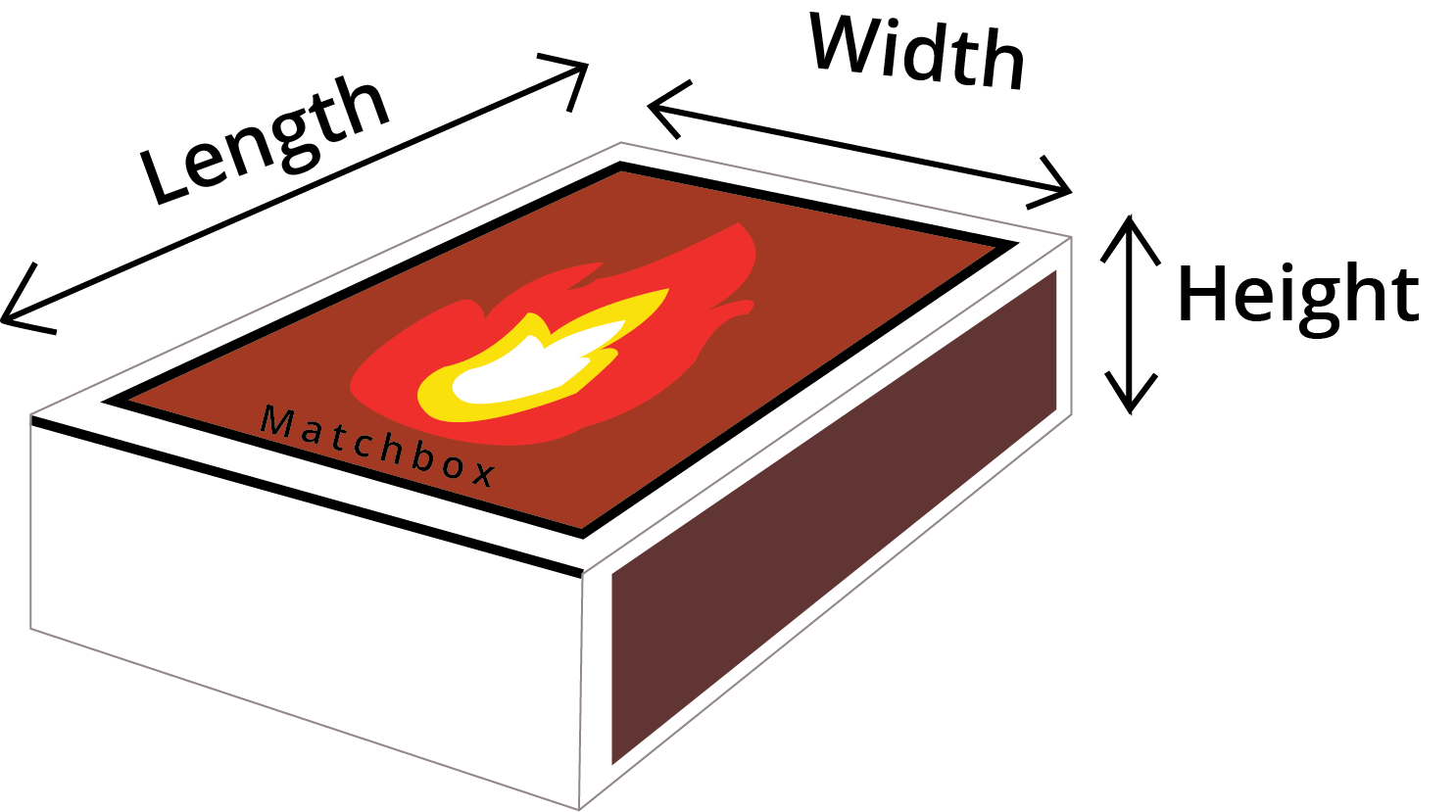Courses
Courses for Kids
Free study material
Free LIVE classes
More

# How Big How Heavy Class 5 Notes CBSE Maths Chapter 14 (Free PDF Download)LIVE
Join Vedantu’s FREE Mastercalss

## NCERT Class 5 Maths Chapter 14 Revision Notes

Now it is easy to learn about volume, mass, and weight with the help of How Big How Heavy Revision Notes prepared by subject experts at Vedantu. NCERT Class 4 Maths Chapter 14 notes help students build a strong foundation about different concepts of volume, length, breadth, mass, weight, and other related terms in the best way.

With the help of these notes, students will find an easy and interesting way of learning through the story-based questions and amazing pictures to help them out. They can understand the topics and aspects of the chapter better with the help of detailed examples. Reading How Big How Heavy Summary will enable them to prepare for their exams in a better way.

Last updated date: 01st Oct 2023
Total views: 145.2k
Views today: 3.45k

## How Big? How Heavy?

• Here, big refers to determining the size of an object, and heavy refers to the object's weight.

• This can be found by calculating the object's volume.

### Measurements

• Kilograms are commonly used to measure the weight of objects.

• Grams (g) and Milligrams (mg) are used to measure the weight of smaller objects (mg).

• Length is measured in Centimeter(cm), Millimeter (mm) and Meter (m).

• One millilitre of water has a volume of 1 cubic centimetre.

### Coin and Marble

• If the weight of two objects is the same, the size and shape of those two quantities do not have to be the same.

• If we take one 5 coins and one marble, both of them weigh the same, but when each of them is placed in a glass with equal amounts of water.  Water will slightly rise in a marble containing water compared to a water-containing coin.

• This is because a marble (which is spherical) has a larger volume than a coin (which is flat), even if they weigh the same.Marble - Coin example

Note: The bigger the object, the more volume or space is occupied by the object.

### Volume

• The capacity of an object is measured by its volume.

• The volume is measured in cubic units such as m3, cm3, and so on.

• For example, a cup's volume is 100 ml if it can carry 100 ml of water up to the top.

### Roughly Comparing the Volumes

• We can roughly compare the volume of one tennis ball to that of five (or more) marble balls.

• This can be calculated by filling two containers with equal amounts of water, placing a tennis ball in one container, and continuing to add marbles to the water in another container until the water level in both containers rises to the same level.Comparing the volume of a Tennis Ball and Marbles

### Length, Width and Height

• The longest dimension of a figure is length, which indicates how long the object is.

• Width is the shorter distance between two points that indicates how broad or wide an object is.

• The height of an object defines how long or deep it is.Cuboid

### Solved Example 1: Find the length, width, height and volume of the matchbox.

Ans: Take a matchbox and a measuring scale.

Measure the length, width and height of the matchbox.

Then calculate its volume = length x width x heightA Matchbox

Here, The length of the matchbox is 5cm long, Its width is 3cm wide and its height is 1cm.

So Its volume =$5\times 3\times 1=15cm^{3}$

### Volume of Cuboid

• A cuboid is a solid geometrical object with 6 faces.

• The number of cubes that can be packed into a cuboidal box is equal to the volume of the cuboidal box.

• Volume of cuboid(V) = length × breadth ×  height

• Volume of cuboid (V)= l × b ×  h

### Solved Example 1: Find the number of cubes that can be packed into the cuboidal box with length=10 cm, breadth=6 cm and height =20 cm.

Ans: length=10 cm, breadth=6 cm and height =20 cm.

Number of cubes packed = Volume of cuboidal box = length x width x height

Number of cubes packed = Volume = 10 x 6 x 20

So, 1200 cubes can be packed.

### Volume of Cube

• A cube is a solid geometrical object with 6 faces.

• All the sides of the cube are equal in length.

• Volume of a cube(V) = length × breadth ×  height

• Volume of a cube(V) = s x s x s  (where s is the side of the cube)

• Volume of a cube(V) = $s^3$

Solved Example 1: Find the volume of the cubical box with length=11 cm, breadth= 11 cm and height =11 cm.

Ans: length=11 cm, breadth=11 cm and height =11 cm.

Volume = length x width x height

Volume = s x s x s

Volume = 11 x 11 x 11

Volume = 1331 $cm^3$

### Counting Coins

• To calculate the weight of the sack, we need to multiply the weight of the sack by 1000. And, to calculate the number of coins, we need to divide the total weight of the sack by the weight of each coin.

• Total weight of sack = Weigh of sack  × 1000 as (1kg =1000g).

• Number of coins = Total Weight of sack $\div$ Weight of each coin.

Solved Example 1: The weight of a 5 rupee coin is 9 g. How many coins are there in a sack of 5 rupee coins if it weighs 27 Kg?

Ans:  The weight of a 5 rupee coin is 9 g, and the weight of a sack is 27Kg.

We know that 1Kg =1000g.

Step1: Find the total weight of sack = Weigh of sack  × 1000 = 27  × 1000 = 2700.

Step2: Write the weight of each coin = 9 g

Step3: Number of coins = Total Weight of sack $\div$ Weight of each coin= 2700 $\div$ 9 = 300

So, the number of coins is 300.

Solved Example 2: A 2 rupee coin weighs 5 g. What is the weight of a sack with 2000 coins?

Ans: Step1: Write the weight of each coin = 5 g

Step2: Write the total number of coins = 2000

Step3: Find the total weight of sack = Total number of coins × weight of each coin = 2000 ×  5 g = 10,000 g.

So, the total weight of the sack is 10,000 g.

## Practice Problem

Question 1. If 5 rupee coins weigh 85 g then how much will 1000 coins weigh?

Question 2. How many cubes can be packed in a box with of length 25 cm, a breadth of 5 cm and a height of 10 cm?

Ans 1. Total weight of 1000 coins  = 85 kg or 85000 g

Ans 2. Total number of cubes = 1250

## Importance of NCERT Class 5 Maths Chapter 14 Revision Notes

• This interesting chapter begins with different sums on figuring out the volume of a particular object. Students will learn the equations to use in order to measure the volume of coins and marbles in a measuring glass.

• Also, students all learn how to figure out the volume of a cube from the length, breadth, and height details.

• The chapter also has different questions where students will be asked to use equations in order to find the length, breadth, and height of a particular matchbox or multiple matchboxes.

• There are activity-based questions with which students will be able to learn the process of measurement better. They can create cubes by folding different pieces of paper.

• You can also learn which has more volume amongst different cubes with the help of calculation processes.

• Proceeding further, students get to learn about cylindrical pipes and learn equations to figure out which pipe would fill more water.

• Furthermore, the chapter also discusses the concept of weight wherein students will understand how much an item weighs. They will also answer different questions using formulas to figure out the weight of an object.

## Benefits of Revision Notes for Class 5 Chapter 14 From Vedantu

Using the notes and worksheets of How Big How Heavy Class 5, students are offered a lot of benefits.

• Learning about the volumes of different objects such as cubes, pipes, and much more.

• Solving easy and complicated questions using different formulas and equations to find out the volume.

• Attempting the making a paper cube activity to learn the measuring process in a better way.

• Precise explanation of equations, formulas, and solving processes to approach different questions and find out the answers.

• Proper guidance from subject experts on finding out the weight and mass of an object in a simplified and easy manner.

• A chance to recall anything that you might have missed in the class.

• Development of problem-solving skills as well as logical thinking and time management abilities.

## Conclusion

Go ahead and download how big and heavy revision notes are from Vedantu. All the questions, examples, and solutions have been crafted by subject experts that can provide the right guidance to you. If you want to score high marks in your exams by preparing this chapter in the best way, these revision notes are the best bet.

## FAQs on How Big How Heavy Class 5 Notes CBSE Maths Chapter 14 (Free PDF Download)

1. How can I measure the volume of a cube?

In order to find the volume of a cube, you have to figure out the length, breadth, and height of the cube in the first place. Multiplying these three values will give you the answer for the volume of the cube.

2. Are revision notes for class 5 chapter 14 beneficial?

Practicing and learning from these revision notes crafted by experts at Vedantu will help you grasp the concepts easily. These notes have been created in a very simple manner which can make the process of preparation very easy.

3. How difficult is Class 5 Maths Chapter 14 How Big How Heavy?

If you don’t have a good understanding of the topics included in chapter 14 of Class 5 maths, it might be a bit difficult for you. But by referring to How Big How Heavy revision notes, you can understand the topics very easily.